# 你确定真正理解联合索引和最左前缀原则？

0收藏

### 介绍

``````CREATE TABLE `teacher` (
`id` int(10) NOT NULL,
`name` char(20) NOT NULL,
PRIMARY KEY (`id`),
KEY `idx_name` (`name`),
) ENGINE=InnoDB DEFAULT CHARSET=utf8;``````

``explain select * from teacher where name = "张三"``

``explain select * from teacher where address = "北京"``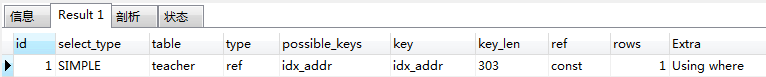### key_len

key_len表示索引使用的字节数，根据这个值，就可以判断索引使用情况，特别是在组合索引的时候，判断所有的索引字段是否都被查询用到

 类型 大小 CHAR 0-255字节 VARCHAR 0-65535字节

char和varchar跟字符编码也有密切的联系latin1占用一个字节，gbk占用2个字节，utf8占用3个字节，utf8mb4占用4个字节（不同字符编码占用的存储空间不同）

char和varchar跟字符编码也有密切的联系

latin1占用一个字节，gbk占用2个字节，utf8占用3个字节，utf8mb4占用4个字节（不同字符编码占用的存储空间不同）

char(n)=n*(utf8mb4=4,utf8=3,gbk=2,latin1=1)

char(n)=n*3+1（允许null，是否为空的标记）

varchar(n)=n*3+2（变长列，记录当前数据存了多少）

varchar(n)=n*3+1（允许null）+2（变长列）

 类型 大小 TINYINT 1字节 INT或INTEGER 4字节 DATE 3字节 TIME 3字节

Not Null=字段本身的长度Null=字段本身的长度+1

### 最左前缀原则

1. 需要查询的列和组合索引的列顺序一致
2. 查询不要跨列

``````CREATE TABLE `people` (
`name` varchar(50) NOT NULL,
`country` varchar(50) NOT NULL,
) ENGINE=InnoDB DEFAULT CHARSET=utf8;``````

``````explain select * from people where name = "jack"
and address = "beijing" and country = "china"``````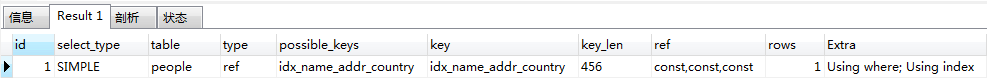type为ref，key_len为456=（50*3+2）*3，联合索引的所有列都使用了

``explain select * from people where name = "jack"``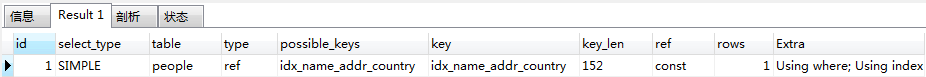type为ref，key_len为152=50*3+2，联合索引只使用了name列

``explain select * from people where address = "beijing"``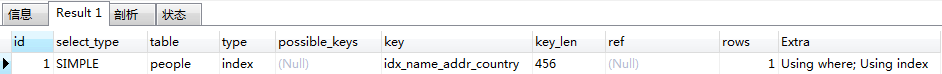type为index，并没有走索引，简单说一下index和ref的区别

index：这种类型表示mysql会对整个该索引进行扫描。要想用到这种类型的索引，对这个索引并无特别要求，只要是索引，或者某个联合索引的一部分，mysql都可能会采用index类型的方式扫描。但是呢，缺点是效率不高，mysql会从索引中的第一个数据一个个的查找到最后一个数据，直到找到符合判断条件的某个索引。所以，上述语句会触发索引扫描

ref：这种类型表示mysql会根据特定的算法快速查找到某个符合条件的索引，而不是会对索引中每一个数据都进行一一的扫描判断，也就是所谓你平常理解的使用索引查询会更快的取出数据。而要想实现这种查找，索引却是有要求的，要实现这种能快速查找的算法，索引就要特定的数据结构。简单说，也就是索引字段的数据必须是有序的，才能实现这种类型的查找，才能利用到索引。

 sql 是否使用索引 where a = x and b = x and c = x 是 where a = x and b = x 是，部分索引 where a = x 是，部分索引 where b = x 否，不包含最左列a where b = x and c = x 否，不包含最左列a

1. 通过有序索引顺序扫描直接返回有序数据
2. Filesort排序，对返回的数据进行排序

``explain select * from people order by name``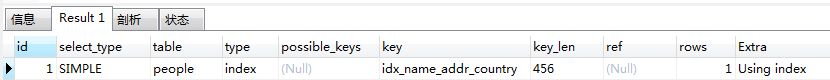Extra列只有Using index，即根据索引顺序进行扫描

``explain select * from people order by address``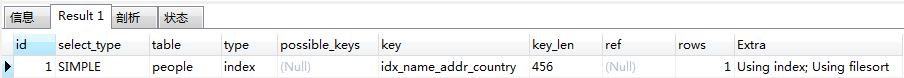Extra列有Using filesort，进行了额外排序

order by 能使用索引排序

``````order by a
order by a,b
order by a,b,c
order by a desc, b desc, c desc
where a = const order by b,c
where a = const and b = const order by c
where a = const and b > const order by b,c``````

order by 不能使用索引进行排序

``````order by b
order by c
order by b, c
order by a asc, b desc, c desc //排序不一致
where g = const order by b,c //丢失a索引
where a = const order by c //丢失b索引
where a = const order by a,d //d不是索引的一部分
where a in (...) order by b,c //范围查询``````

### 联合索引特性帖子
视频
声望
粉丝
最近发布
热门推荐
社区精华内容